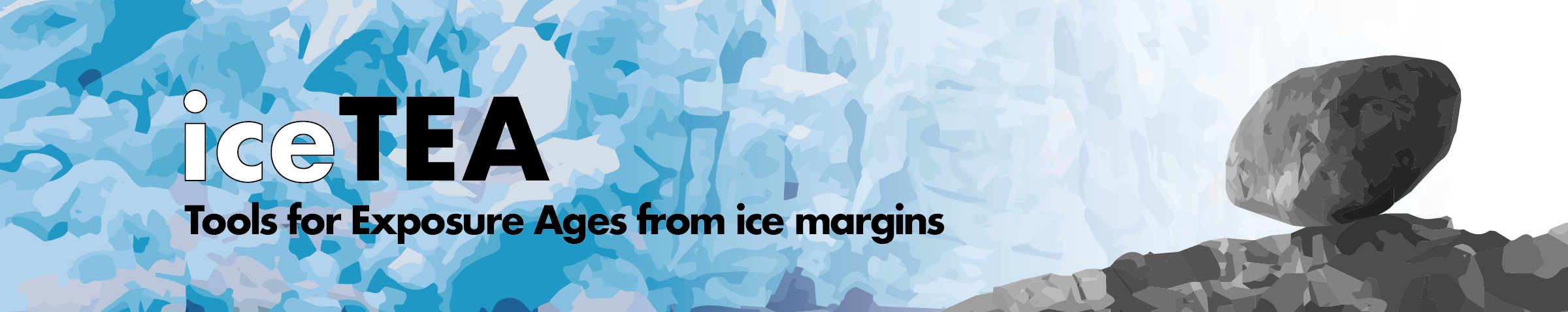## Documentation

iceTEA is an online and MATLAB© based suite of tools for plotting and analysing cosmogenic-nuclide surface-exposure data from former glacier and ice sheet margins.

Check out the paper on iceTEA for full descriptions of the tools and examples of how they can be used: Jones et al., 2019, Quaternary Geochronology (preprint version here).

Currently these tools can only be used for 10Be and 26Al concentrations and exposure ages, but we intend to expand the tools so that they can be used with 3He, 14C, 21Ne and 36Cl data.

Three of the tools perform exposure age calculations, which are based on the framework of CRONUScalc (Marrero et al., 2016).

### Code

iceTEA also exists as MATLAB© code with easy-to-use front-end scripts for each tool. This code has some added functionality and, for more exerienced MATLAB© users, allows tools to be modified for specific and more complex needs.

Description of functions:  iceTEA manual for MATLAB .

### Input data

Sample data is required as a Microsoft© Excel© (.xlsx) or comma-separated values (.csv) spreadsheet, or in a tab-delimited text file (.txt). Data should be in one of two different forms: Standard or Complex.

Standard input

This is for use with all of the tools except the ‘Plot Isotope Concentrations’ tool, and requires either 15 or 22 columns of sample information:
1. Sample name
2. Latitude (decimal degrees)
3. Longitude (decimal degrees)
4. Elevation (m asl)
5. Pressure (hPa) (zero if not known)
6. Elevation uncertainty (m) (zero if not known, in which case 5 m is used)
7. Relative position (distance from terminus, km; elevation above ice, m) (zero or NaN if not relevant or known)
8. Sample thickness (cm)
9. Bulk density (g cm-3)
10. Shielding factor for terrain, snow, etc. (unitless)
11. Sample 10-Be concentration (atoms of 10Be/g) (zero if not measured)
12. Sample 10-Be concentration 1 sigma uncertainty (atoms of 10Be/g) (zero if not measured)
13. Sample 26-Al concentration (atoms of 26Al/g) (zero if not measured)
14. Sample 26-Al concentration 1 sigma uncertainty (atoms of 26Al/g) (zero if not measured)
15. Year the sample was collected (calendar year)

Optional information that is required for importing previously calculated ages (zeros can be used if the nuclide was not measured):
16. Sample 10-Be exposure age (mean; years)
17. Sample 10-Be exposure 1 sigma uncertainty (internal; years)
18. Sample 10-Be exposure 1 sigma uncertainty (external; years)
19. Sample 26-Al exposure age (mean; years)
20. Sample 26-Al exposure 1 sigma uncertainty (internal; years)
21. Sample 26-Al exposure 1 sigma uncertainty (external; years)
22. Scaling model used (i.e. 'DE', 'DU', 'LI', 'ST', 'Lm', 'LSD', 'LSDn')

Complex input

The sample data for the ‘Plot Isotope Concentrations’ tool should be in 17 columns:
1. Sample name
2. Latitude (decimal degrees)
3. Longitude (decimal degrees)
4. Elevation (m asl)
5. Pressure (hPa) (zero if not known)
6. Relative position (distance from terminus, km; elevation above ice, m)
7. Sample thickness (cm)
8. Bulk density (g cm-3)
9. Shielding factor for terrain, snow, etc. (unitless)
10. Sample 10-Be concentration (atoms of 10Be/g)
11. Sample 10-Be concentration 1 sigma uncertainty (atoms of 10Be/g)
12. Sample 26-Al concentration (atoms of 26Al/g)
13. Sample 26-Al concentration 1 sigma uncertainty (atoms of 26Al/g)
14. Top depth of sample (cm) (the surface is zero)
15. Bottom depth of sample (cm)
16. Final mineral weight (g)
17. Year the sample was collected (calendar year)